# Sample Data: Scallop Abundance

Locations of scallop samples in the Atlantic Ocean, annotated with marks including the total number of scallops caught, the number of pre-recruit scallops, and the number of recruit scallops

## Details

Locations of scallop samples in the Atlantic Ocean in the observation region which is the convex hull of the sample locations, annotated with marks including the total number of scallops caught, the number of pre-recruit scallops (<70mm shell length), and the number of recruit scallops (>=70mm shell length).

## Examples

### Basic Examples (2)

Retrieve the data:

 In:=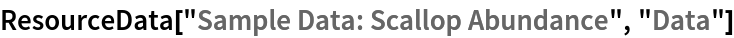Out=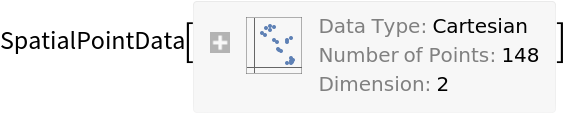Summary of the spatial point data:

 In:=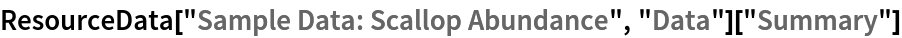Out=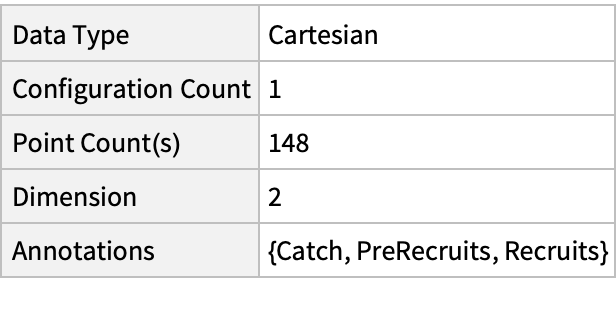### Visualizations (3)

Plot the spatial point data:

 In:=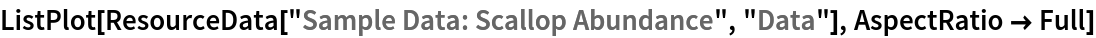Out=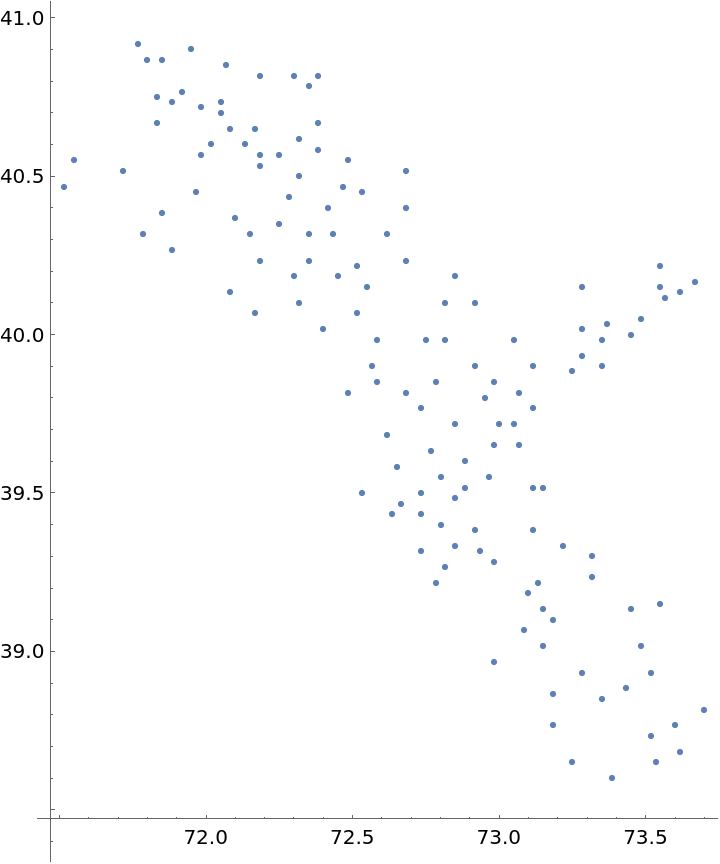Visualize the data with some annotations:

 In:=Out=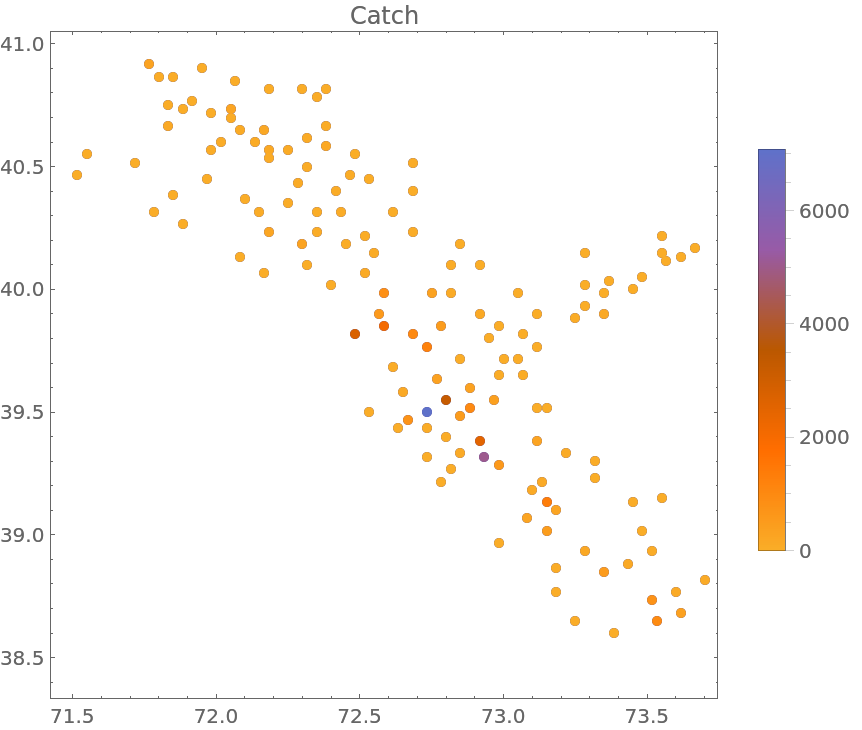Visualize the smooth point density of the data:

 In:=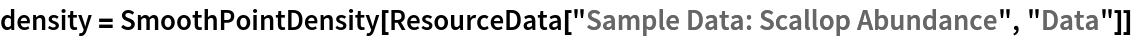Out=In:=Out=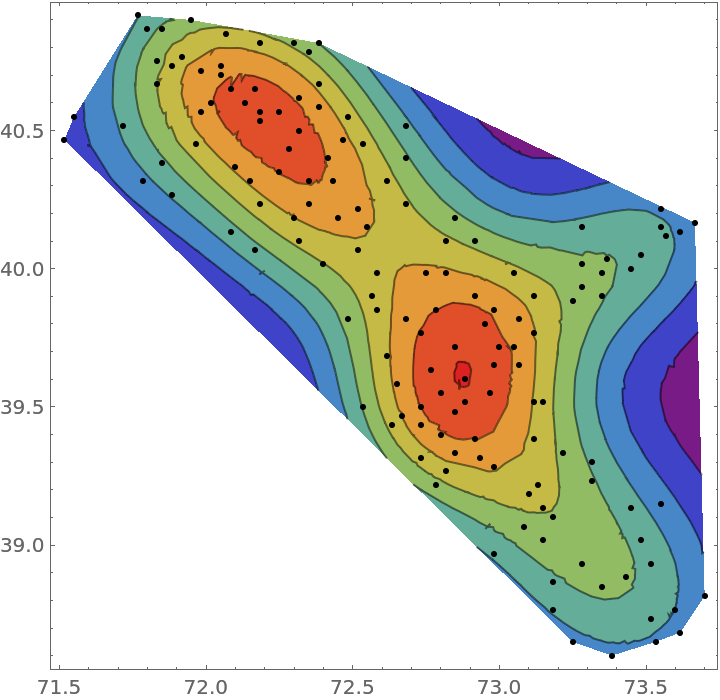Gosia Konwerska, "Sample Data: Scallop Abundance" from the Wolfram Data Repository (2021)